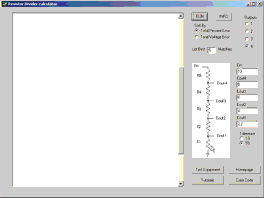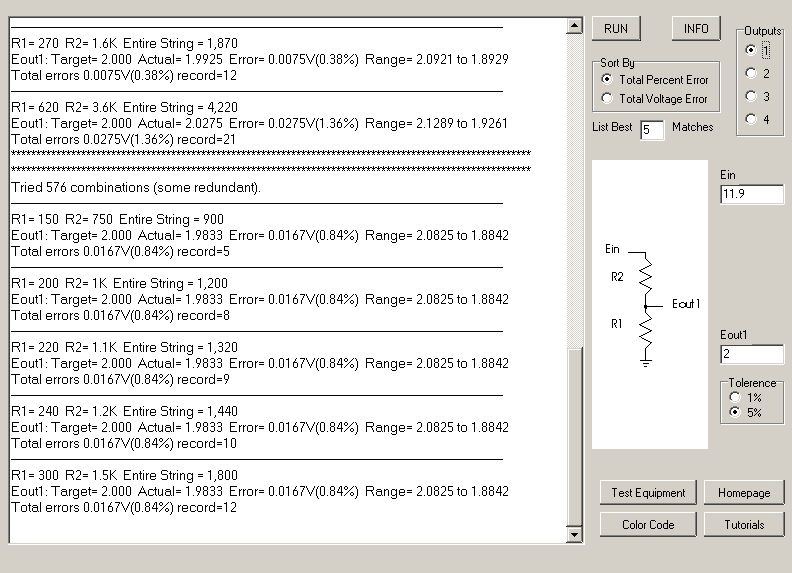## Disclaimer

This information HAS errors and is made available WITHOUT ANY WARRANTY OF ANY KIND and without even the implied warranty of MERCHANTABILITY or FITNESS FOR A PARTICULAR PURPOSE. It is not permissible to be read by anyone who has ever met a lawyer or attorney. Use is confined to Engineers with more than 370 course hours of engineering.
If you see an error contact:
+1(785) 841 3089
inform@xtronics.com

# Resistor Dividers

## Resistor Picker Software

Calculates optimal stock values to use in a resistor string. Sorts output via Total percentage error of the outputs or Total Voltage error. Switches between 5% and 1% stock values. Setting for number of matches to calculate. Tests all permutations of appropriate values.Screenshot1Screenshot2

### Sample output

``` **********************************************************************
**********************************************************************
Tried 7962624 combinations (some redundant).
---------------------------------------------------------------------------------------------------------------------------
R1= 220 R2= 180 R3= 100 R4= 300 R5= 200
Eout1: Target= 2.2 Actual= 2.2 Center Error= 0V(0%) Eout Range= 2.31 to 2.09
Eout2: Target= 4 Actual= 4 Center Error= 0V(0%) Eout Range= 4.2 to 3.8
Eout3: Target= 5 Actual= 5 Center Error= 0V(0%) Eout Range= 5.25 to 4.75
Eout4: Target= 8 Actual= 8 Center Error= 0V(0%) Eout Range= 8.4 to 7.6
Total errors 0V(0.00%) record=9
---------------------------------------------------------------------------------------------------------------------------
R1= 240 R2= 200 R3= 110 R4= 330 R5= 220
Eout1: Target= 2.2 Actual= 2.1818 Center Error= 0.0182V(0.83%) Eout Range= 2.291 to 2.073
Eout2: Target= 4 Actual= 4 Center Error= 0V(0%) Eout Range= 4.2 to 3.8
Eout3: Target= 5 Actual= 5 Center Error= 0V(0%) Eout Range= 5.25 to 4.75
Eout4: Target= 8 Actual= 8 Center Error= 0V(0%) Eout Range= 8.4 to 7.6
Total errors 0.0182V(0.83%) record=10
---------------------------------------------------------------------------------------------------------------------------
R1= 1.1K R2= 910 R3= 510 R4= 1.5K R5= 1K
Eout1: Target= 2.2 Actual= 2.1912 Center Error= 0.0088V(0.4%) Eout Range= 2.301 to 2.082
Eout2: Target= 4 Actual= 4.004 Center Error= 0.004V(0.1%) Eout Range= 4.204 to 3.804
Eout3: Target= 5 Actual= 5.0199 Center Error= 0.0199V(0.4%) Eout Range= 5.271 to 4.769
Eout4: Target= 8 Actual= 8.008 Center Error= 0.008V(0.1%) Eout Range= 8.408 to 7.608
Total errors 0.0406V(1.00%) record=2
```

 Top Page wiki Index

Email